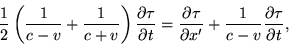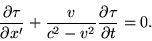Algebra problem

Hi following a link on another thread I began to read On The Electrodynamics Of Moving Bodiesshould equalbut I end up with a minus instead of a plus between the two fractions(in the last equation).

I've gone through my calculations but I cannot see where I've gone wrong.

I could post my working out if neccessary but it might be hard to read in this format

Hurkyl
Staff Emeritus
Gold Member
I assume you got this far on the coefficient of [pard]&tau/[pard]t

(1/2) * (1/(c-v) - (1/c+v))

Collecting fractions:

(1/2) * ((c+v) - (c-v)) / ((c-v)*(c+v))
(1/2) * (2v) / (c^2 - v^2)
v / (c^2 - v^2)

Hurkyl

(edit: fixed tags)

Last edited:
HallsofIvy
Homework Helper
It's almost impossible to catch your own error and the sillier the error is the harder it is to find it!

In the first formula you have 1/(c-v)+ 1/(c+v) on one side. You get common denominators, of course, and have ((c+v)+(c-v))/(c^2- v^2)= 2c/(c^2- v^2) which is then multiplied by 1/2: c/(c^2- v^2)

On the other side of the same formula you have 1/(c-v). Subtracting c/(c^2- v^2) from both sides: 1/(c-v)-c/(c^2- v^2)= c/(c^2-v^2)- (c+v)/(c^2-v^2)= v/(c^2- v^2).

Did you move the fraction to the wrong side?

After I got c/(c^2- v^2) on left side I moved the
1/(c-v)over to isolate [pard]T/[pard]x over on the right then
removed [pard]T/[pard]t from the brackets to get the fraction v/(c^2- v^2).

I them moved the whole lot across to put it equal to zero

Integral
Staff Emeritus
Gold Member
I am not sure if http://home.attbi.com/~rossgr1/Specialrel.PDF [Broken] addresses you exact problem, but you may find it smooths your way to the final DE.

Last edited by a moderator:
Ok that PDF works it out a different way but, even now, things don't look right

From the bottom of the second page

We have "Now recombining the RHS and LHS we have:"

1/2 ((1/c+v)+(1/c-v))[pard]T/[pard]t = [pard]T/[pard]x +(1/c+v)([pard]T/[pard]t)

Right, fine and dandy

BUT, when I collate the terms I get:

1/2((1/c-v)-(1/c-v)([pard]T/[pard]t)=[pard]T/[pard]x

NOT

1/2((1/c+v)-(1/c-v)([pard]T/[pard]t)=[pard]T/[pard]x

So when it comes to multiple both sides with -1 I still get a sign discrepancyI'm going to start to cry real soon...

Integral
Staff Emeritus
Gold Member
I am getting the same result as you, let me look at this to see if I can sort out what happened. It has been a year since I wrote that PDF up, so I need to take some time to get back into the material.

Integral
Staff Emeritus
Gold Member
Well, since I could not find an obvious error in my PDF, I went back to the source. There is where I found MY error. Compare my RHS to Einstein's in my starting equaition. You will see that I have a 1/(c+v) where he has a 1/(c-v). If you replace my RHS term with the correct one it all comes out in the end. Since I worked all this out on paper then transcriped to MS Word editor and PDF, I will claim it as a typo.

My apology, and thanks all at the same time. I have posted that PDF several times, here and other places over the past year. It looks like someone finally read it!

Alleluia

I thought I was going mad.

It's a good paper, I bet lots of people read it but I bet they rarely actually follow the math.

Thanks again.

Integral
Staff Emeritus